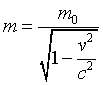QUESTION #161

# What is rest mass? How to explain it especially to the high school students?

One of the consequences of Einstein's special theory of relativity (1905) is that the mass of an object increases with its velocity relative to the observer. When an object is at rest (relative to the observer), it has the usual (inertial = tendency to resist an applied force) mass that we are all familiar with. This is called the 'rest mass' of the object.

As the speed of the object is increased, the inertial mass of the object also increases. For speeds significantly less than the speed of light, the increase in mass is nearly imperceptible, but as the speed of light is approached, the mass starts to increase very rapidly toward infinity. Theoretically, the mass would become infinite if the object could be accelerated all the way to the speed of light. However, because the acceleration of an object in response to a given force is inversely proportional to its inertial mass, as the speed of light is approached the force required actually to reach the speed of light also becomes infinite. Therefore, it is impossible actually to accelerate an object with non-zero rest mass to the speed of light.

Another way of expressing the fact that a massive object cannot be accelerated to the speed of light is through the concept of energy. That is, an infinite amount of energy would have to be expended, via the accelerating force, to reach the speed of light.

So, quite simply, the 'rest mass' of an object is the inertial mass that an object has when it is at rest.
Answered by: Warren Davis, Ph.D., President, Davis Associates, Inc., Newton, MA USA

Mass measures the amount of inertia an object has, with inertia defined as the resistance the object offers to a change in its state of motion. While we usually think of mass as being constant for an object, Relativity tells us that energy and mass are interchangeable. Any object in motion has, by definition, Kinetic Energy. That energy has the effect of increasing the object's mass, so the MINIMUM mass of an object is its mass when stationary, or its REST MASS.

Mathematically, an object's true mass is given by the expression:where m0 is its mass at rest, v is its velocity, c is the speed of light.

You can see that when v=0, square root of (1-0) equals 1 and m = m0. As velocity increases, (1-v2/c2) becomes less than 1, so square root of (1-v2/c2) also becomes less than 1, and m becomes greater than m0. In fact, as v gets close to c, the square root of (1-v2/c2) value becomes so small that when divided into m0, m represents an inertia too great to overcome and actually attaining a velocity equal to c becomes impossible. At that point, dividing m0 by 0 gives an infinite mass.

Since c is over 186,000 miles/second, we are not accustomed to dealing with objects travelling at velocities high enough to have a mass noticeably greater than their rest mass. Experiments with subatomic particles, however, confirm Relativity's prediction of increasing mass at speeds near c. Devices from particle accelerators to TV picture tubes must account for mass increase in order to work properly.
Answered by: Paul Walorski, B.A. Part-time Physics Instructor